Example Questions

Example Question #31 : Statistics

Fred received an 82, 85, and 95 on his first 3 tests of the semester. All tests are out of 100 points. What must Fred score on the fourth and final test to have an average of 90 for the class?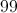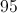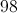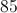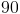Explanation:

The answer isThe total points for the four tests are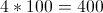points. 90% of 400 is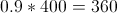. Therefore, we know that Fred must have a total of 360 points from his four tests. We can set up the equation: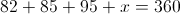This simplifies to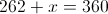.

Subtract 262 from both sides to determine that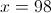.

Example Question #32 : Statistics

If Jamison scored a 68, 88, 82, and 56 on each of his exams, what score must he get on his final test, weighted equally, to recieve a passing average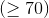?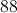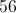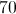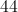Explanation:

This question at first may seem like a daunting amount of arithmetic, but it should be an easy question or essentially free points on the ACT. The problem is set up as a simple equation of averages, shown by: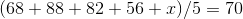Whereis the score of his fifth test.

After adding each of the four tests together, we have: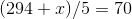Multiple both sides by 5,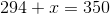Then solve for.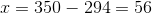Do not be intimidated by questions like these, they are straight-forward and only involve arithmetic.

Example Question #31 : How To Find Arithmetic Mean

If the average of A, B, and C is 50, which of the following expressions represents the average of A, B, C, and D?

[150 - (A + B + C)] / 4

(50 + D) / 4

(150 + D) / 3

(150 + D) / 4

(150 + D) / 4

Explanation:

We take the average 50 and multiply it by 3 (the number of terms in the set) to get the total sum of the initial set. Then you take the total sum and add D and divide by the numbers of terms in the new set.

Example Question #32 : How To Find Arithmetic Mean

Calculate the arithmetic mean of the following set of numbers: 54, 35, 50, 49, 54, 65, 82, 54, and 25.

51

52

53

54

52

Explanation:

To solve this question, you must know that the mean is the sum of the values divided by the number of values, in this case: 468/9=52. It is not 54, which is the median (the most commonly occurring number of the set).

Example Question #31 : Arithmetic Mean

In a theatre there are 600 people. Out of this, 100 males have an average age of 50 and 500 females have an average age of 30. To the nearest whole year, what is the average age of the town’s entire population?

34

32

40

33

43

33

Explanation:

To find the average age, compute:

100 * 50 + 500 * 30= 5000+ 15000= 20000. Then divide by the total amount of people that are at the theatre: 20000/600= 33.3=33.

Example Question #31 : Arithmetic Mean

Jack earned $10 on Tuesday. On each following day, through Satuday, he earned$3 more than the preceding day. What were his mean earnings for the five-day period, Tuesday through Saturday?

$16$5

$80$15

$50 Correct answer:$16

Explanation:

Jack earned $10 on Tuesday,$13 on Wednesday, $16 on Thursday,$19 on Friday, and $22 on Saturday. This is a total of$80. Divide that by the total number of days, 5, and the mean is $16. Example Question #31 : Arithmetic Mean Fred has had three algebra tests so far and has one left before he gets a final grade. He wants an "A" in the class, and so he needs at least a 90% average test grade to get an "A". His previous test scores have been 96%, 86%, and 94%. What is the lowest score he can get on his fourth test to get an "A" in the class? Possible Answers: 85% 84% 94% 90% 88% Correct answer: 84% Explanation: The following equation can be set up in order to solve for the score on the fourth test, x. The left side of the equation is the average of all of his tests. This is then set equal to 90%, the average test score which guarantees him an A: (96+86+94+x) / 4 = 90. Solving for x, we get x = 84%. Example Question #31 : Arithmetic Mean Mark has a job mowing lawns for some of the people in his neighborhood. If Mark gets paid$30 per lawn, and it takes him 40 minutes to mow a lawn, what is his average hourly pay if he typically spends 4 hours total mowing lawns?

$25/hr$30/hr

$35/hr$40/hr

$45/hr Correct answer:$45/hr

Explanation:

This question requires us to do a few things. First, we must figure out how many lawns Mark mows in 4 hours.

4 hours x 60 minutes/hr = 240 minutes.

240 minutes ÷ 40 minutes/lawn = 6 lawns.

6 lawns x $30/lawn =$180

So, he made $180 in a matter of 4 hours.$180 ÷ 4 hours = \$45/hr

Example Question #51 : Data Analysis

Jenny just got her spring report card.  She earned 3 As, 2 Bs and one C.  If the values assigned to grades are 4 points for an A, 3 points for a B, and 2 points for a C, what is her GPA, rounded to the nearest tenth?

3.1

3.7

3.0

3.5

3.3

3.3

Explanation:

GPA is the average of the grade points.

GPA = (3*4 + 2*3 + 1*2) / 6 = 3.33

Example Question #33 : Arithmetic Mean

A student has scored 93, 87, and 94 on three of his four exams. In order to maintain a 90 average or above in the class, what is the lowest this student may score on the fourth exam?

78

89

88

86

92

86

Explanation:

In order to have an average of 90 over four exams, that means the sum of all the students scores must total to at least 360.

So far, the student has a total sum of 274 points.

360 - 274 yields 86, the minimum score needed to maintain an average of 90 or higher